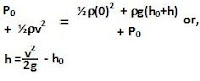## Saturday, May 9, 2009

### Irodov Problem 1.320At the surface level of the water within the tube the velocity of the water is v and directed vertically upwards (this will be same as the velocity of the water at the surface in the rest of the area). Let the atmospheric pressure be Po. At the maximum height the velocity of the water is 0 and so we can use the Bernoulli equation to determine the maximum height as,Alan said...

As for this problem, could you please the equations you wrote?

Why is p0 the difference betweeen preasurres? Does that imply that in the pipe at water level, the preassure iz 0 (and from whare does thet come from)?

Why did you ignore all the other factors of the Bernulli equation?

Krishna Kant Chintalapudi said...

Dear Alan,

i) Po is the atmospheric pressure and this is in both side of the equation.

ii)Height at the surface of the water is taken to be 0 and so the potential energy term on the LHS is absent.

iii) rest are the kinetic energy terms